Do you like the article?
Share it with others —
Use new possibilities of MetaTrader 5

#### Similar articles# How to analyze the trades of the Signal selected in the chart

MetaTrader 5Examples | 30 July 2018, 10:0717 0870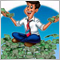### Introduction

New signals, free-of-charge or fee-based, appear in the Signals service on a permanent basis. The MetaTrader team took care that the service could be used without logging out of the terminal. All that is left to do is choosing the very signal that would produce the highest profits at acceptable risks. This problem has been discussed since long ago. The method of automatically selecting signals by the specified criteria  has already been proposed. However, the conventional wisdom says that a picture is worth a thousand words. In this paper, I propose to study and analyze the history of trades on the signal selected in a symbol chart. Perhaps, this approach would let us better understand the strategy of trading and estimate risks.

### 1. Setting the goals for our future work

I hear you cry: 'Why recreate the wheel, if the terminal has already provided the possibility of showing the trading history in the chart? I mean, it is sufficient to select the signal you want and press the button in the terminal.'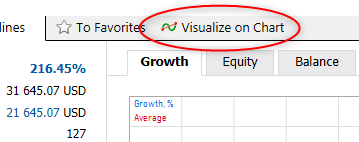Upon that, new windows will open in the terminal, according to the number of the symbol signals used, with markings on the trades made. Of course, paging the charts and searching trades in them are quite laborious activities. Moreover, trades made in different charts may coincide in time, and you cannot see that when analyzing each chart separately. At this stage, we are going to try and automate a part of our work.

In order to identify the symbol that we need for analyzing the charts obtained, we must understand clearly what final results we need. Here are the basic items of what I would like to finally have:

• Seeing how evenly the signal works on different symbols;
• Knowing how the load on the deposit is distributed and how many positions can be opened simultaneously;
• If the signal opens several positions simultaneously, whether they are hedging ones or intensify the loads on the deposit;
• At what moments and on what symbols the largest drawdowns occur; and
• At what moments the largest profit is achieved.

#### 2.1. Class for keeping information on the order

So, we select the desired signal and display its trade history in the chart. Then collect initial data that we are going to analyze after that. To record information on each individual order, we create class COrder based on class CObject. In the variables of this class, we save the order ticket, trade type and lot size, trade price, operation type (input / output), order opening time, and, of course, the symbol.

```class COrder : public CObject
{
private:
long                 l_Ticket;
double               d_Lot;
double               d_Price;
ENUM_POSITION_TYPE   e_Type;
ENUM_DEAL_ENTRY      e_Entry;
datetime             dt_OrderTime;
string               s_Symbol;

public:
COrder();
~COrder();
bool                 Create(string symbol, long ticket, double volume, double price, datetime time, ENUM_POSITION_TYPE type);
//---
string               Symbol(void)   const {  return s_Symbol;     }
long                 Ticket(void)   const {  return l_Ticket;     }
double               Volume(void)   const {  return d_Lot;        }
double               Price(void)    const {  return d_Price;      }
datetime             Time(void)     const {  return dt_OrderTime; }
ENUM_POSITION_TYPE   Type(void)           {  return e_Type;       }
ENUM_DEAL_ENTRY      DealEntry(void)const {  return e_Entry;      }
void                 DealEntry(ENUM_DEAL_ENTRY value) {  e_Entry=value; }
//--- methods for working with files
virtual bool         Save(const int file_handle);
//---
//--- method of comparing the objects
virtual int          Compare(const CObject *node,const int mode=0) const;
};
```

Along with the data access function, we add to the orders class the functions of working with files to save and subsequently read the data, as well as the function of comparing to the similar object, which we will need for sorting the orders.

To compare to orders, we will need re-writing virtual function Compare. This is the function of the basic class, developed for comparing to objects CObject. Therefore, the link to object CObject and the sorting method are passed to its parameters. We are going to sort our orders in only one direction, i.e., in the execution date ascending order, so we won't use parameter 'mode' in the function code. However, for working with object COrder obtained via the link, we first have to reduce it to the relevant type. After that, we compare the dates of the obtained order and of the current order. If the obtained order is older, return "-1". If it is newer, then return "1". If the dates of executing the orders are equal, the function will return "0".

```int COrder::Compare(const CObject *node,const int mode=0) const
{
const COrder *temp=GetPointer(node);
if(temp.Time()>dt_OrderTime)
return -1;
//---
if(temp.Time()<dt_OrderTime)
return 1;
//---
return 0;
}
```

#### 2.2. Collecting information from the charts

For working with orders, we create class COrdersCollection based on class CArrayObj. Information will be collected and processed in it. To store the data, we are going to declare an object instance for directly working with a specific order and an array for storing the list of symbols used. We are going to store the array of orders using the basic-class functions.

```class COrdersCollection : public CArrayObj
{
private:
COrder            *Temp;
string            ar_Symbols[];

public:

COrdersCollection();
~COrdersCollection();
//--- Initialization
bool              Create(void);
int               Symbols(string &array[]);
bool              GetPosition(const string symbol, const datetime time, double &volume, double &price, ENUM_POSITION_TYPE &type);
datetime          FirstOrder(const string symbol=NULL);
datetime          LastOrder(const string symbol=NULL);
//--- Obtaining timeseries
bool              GetTimeSeries(const string symbol, const datetime start_time, const datetime end_time, const int direct,
double &balance[], double &equity[], double &time[], double &profit, double &loss,int &long_trades, int &short_trades);
//---
void              SetDealsEntry(void);
};
```

Function 'Create' is directly responsible for collecting data. Within the method body, we are going to arrange a loop for searching in all the charts opened in the terminal. We are going to search for graphic objects like OBJ_ARROW_BUY and OBJ_ARROW_SELL in each chart.

```bool COrdersCollection::Create(void)
{
long chart=ChartFirst();
while(chart>0)
{
int total_sell=ObjectsTotal(chart,0,OBJ_ARROW_SELL);
{
chart=ChartNext(chart);
continue;
}
```

If the object is found in the chart, then we add the chart symbol into our symbol array (however, we precheck whether such symbol is not among those already saved).

```      int symb=ArraySize(ar_Symbols);
string symbol=ChartSymbol(chart);
bool found=false;
for(int i=0;(i<symb && !found);i++)
if(ar_Symbols[i]==symbol)
{
found=true;
symb=i;
break;
}
if(!found)
{
if(ArrayResize(ar_Symbols,symb+1,10)<=0)
return false;
ar_Symbols[symb]=symbol;
}
```

Then we arrange collecting information on trades from the chart into a data array. Note: The only trade information source we have is the graphical object. From the object parameters, we can only get the time and price of the trade. We will have to take all other details from the object name that represents a text string.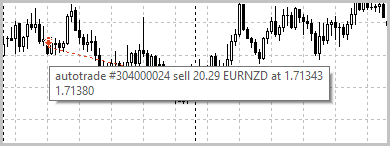In the picture, you can see that the object name comprises all the data on the trade, divided by spaces. Let us use this observation and divide the string by spaces into an array of string elements. Then we will reduce the information from the relevant element to a desired data type and save it. Upon collecting the information, we go to the next chart.

```      int total=fmax(total_buy,total_sell);
for(int i=0;i<total;i++)
{
{
datetime time=(datetime)ObjectGetInteger(chart,name,OBJPROP_TIME);
StringTrimLeft(name);
StringTrimRight(name);
StringReplace(name,"#","");
string split[];
StringSplit(name,' ',split);
Temp=new COrder;
if(CheckPointer(Temp)!=POINTER_INVALID)
{
}
}
//---
if(i<total_sell)
{
string name=ObjectName(chart,i,0,OBJ_ARROW_SELL);
datetime time=(datetime)ObjectGetInteger(chart,name,OBJPROP_TIME);
StringTrimLeft(name);
StringTrimRight(name);
StringReplace(name,"#","");
string split[];
StringSplit(name,' ',split);
Temp=new COrder;
if(CheckPointer(Temp)!=POINTER_INVALID)
{
if(Temp.Create(ar_Symbols[symb],StringToInteger(split),StringToDouble(split),StringToDouble(split),time,POSITION_TYPE_SELL))
}
}
}
chart=ChartNext(chart);
}
```

There is no information in graphical marks on whether a position was opened or closed for each trade. This is why this field is still unfilled when saving the information on trades. Now, upon having collected all marks from the chart, we will add lacking data by calling function SetDealsEntry.

```   SetDealsEntry();
//---
return true;
}
```

To avoid duplication of trades in our database, we will re-write function Add, adding to it checking the order availability by ticket.

```bool COrdersCollection::Add(COrder *element)
{
for(int i=0;i<m_data_total;i++)
{
Temp=m_data[i];
if(Temp.Ticket()==element.Ticket())
return true;
}
//---
}
```

To arrange the types of operations in the trades, we will create function SetDealsEntry. At its beginning, we call the basic class sorting function. The arrange a loop to search in all symbols and the trades on each of them. Algorithm of identifying the operation type is simple. If, as of the operation, there is no open position or there is one in the same direction as the trade, then we identify this operation as entry into a position. If the operation is opposite to the existing position, then its lot size is first used to close the open position, and the rest opens a new position (similar to the netting system of MetaTrader 5).

```COrdersCollection::SetDealsEntry(void)
{
Sort(0);
//---
int symbols=ArraySize(ar_Symbols);
for(int symb=0;symb<symbols;symb++)
{
double volume=0;
ENUM_POSITION_TYPE type=-1;
for(int ord=0;ord<m_data_total;ord++)
{
Temp=m_data[ord];
if(Temp.Symbol()!=ar_Symbols[symb])
continue;
//---
if(volume==0 || type==Temp.Type())
{
Temp.DealEntry(DEAL_ENTRY_IN);
volume=NormalizeDouble(volume+Temp.Volume(),2);
type=Temp.Type();
}
else
{
if(volume>=Temp.Volume())
{
Temp.DealEntry(DEAL_ENTRY_OUT);
volume=NormalizeDouble(volume-Temp.Volume(),2);
}
else
{
Temp.DealEntry(DEAL_ENTRY_INOUT);
volume=NormalizeDouble(volume-Temp.Volume(),2);
type=Temp.Type();
}
}
}
}
}
```

#### 2.3. Creating the timeseries of the balance and funds on each symbol

To build balance and funds charts for each symbol later, we will need creating timeseries calculating these parameters within the entire period analyzed. When analyzing, we would preferably be able to change the period under analysis. This will help study how the signal works at limited time intervals.

We will calculate the timeseries in function GetTimeSeries. In its parameters, we will specify the symbol and starting and ending times of the period to be analyzed, as well as trading directions, to track long and short positions. The function will return three timeseries: Balance, funds, and time marks. Moreover, it will return statistics on the symbol over the period analyzed: Profits, losses, long trades, and short trades.

Looking ahead, I would like to focus your attention on the fact that the array for the timeseries of time marks is defined as double. This small trick is a lesser-evil solution. Then we will build the charts for balance and funds, using standard class CGraphic that only accepts double-type arrays.

At the beginning of the function, we will reset to zero the variables for collecting statistics, check the symbol for its correctness, and get the price of one point of price changing.

```bool COrdersCollection::GetTimeSeries(const string symbol,const datetime start_time,const datetime end_time,const int direct,double &balance[],double &equity[], double &time[], double &profit, double &loss,int &long_trades, int &short_trades)
{
profit=loss=0;
//---
if(symbol==NULL)
return false;
//---
if(tick_value==0)
return false;
```

To build timeseries, we will use the quotes of a symbol with timeframe M5, i.e., we should download them. But note: The quotes we have requested for may have not been formed yet. Here we have another trick: We won't loop the operations and wait until the data is loaded, since that would completely stop executing the program and may slow down the terminal when used in indicators. Upon the first unsuccessful call, we will quit the function. However, before that, we will create a user-defined event that would later re-call the data updating function.

```   ENUM_TIMEFRAMES timeframe=PERIOD_M5;
//---
double volume=0;
double price=0;
ENUM_POSITION_TYPE type=-1;
int order=-1;
//---
MqlRates rates[];
int count=0;
count=CopyRates(symbol,timeframe,start_time,end_time,rates);
{
return false;
}
```

Upon loading the quotes, we will align the size of the timeseries arrays with the size of the quotes loaded.

```   if(ArrayResize(balance,count)<count || ArrayResize(equity,count)<count || ArrayResize(time,count)<count)
return false;
ArrayInitialize(balance,0);
```

Then we will arrange a loop to collect the information for timeseries. We will identify the operations made on each bar. If this is a position opening operation, then we will increase the lot size of the current position and re-calculate the average open price. If this is a position closing operation, we will re-calculate the profits/losses of the operation, add the obtained value to the balance change on the current bar, and reduce the lot size of the current position. Then, for the lot size of the position that has not been closed by the time of the bar closing, we calculate the unclosed profits/losses and save the obtained value in the funds changing on the bar being analyzed. Upon search in the entire history, we exit the function.

```   do
{
order++;
if(order<m_data_total)
Temp=m_data[order];
else
Temp=NULL;
}
while(CheckPointer(Temp)==POINTER_INVALID && order<m_data_total);
//---
for(int i=0;i<count;i++)
{
while(order<m_data_total && Temp.Time()<(rates[i].time+PeriodSeconds(timeframe)))
{
if(Temp.Symbol()!=symbol)
{
do
{
order++;
if(order<m_data_total)
Temp=m_data[order];
else
Temp=NULL;
}
while(CheckPointer(Temp)==POINTER_INVALID && order<m_data_total);
continue;
}
//---
if(Temp!=NULL)
{
if(type==Temp.Type())
{
price=volume*price+Temp.Volume()*Temp.Price();
volume+=Temp.Volume();
price=price/volume;
switch(type)
{
break;
case POSITION_TYPE_SELL:
break;
}
}
else
{
if(i>0 && (direct<0 || direct==type))
{
double temp=(Temp.Price()-price)*tick_value*(type==POSITION_TYPE_BUY ? 1 : -1)*MathMin(volume,Temp.Volume());
balance[i]+=temp;
if(temp>=0)
profit+=temp;
else
loss+=temp;
}
volume-=Temp.Volume();
if(volume<0)
{
volume=MathAbs(volume);
price=Temp.Price();
type=Temp.Type();
switch(type)
{
break;
case POSITION_TYPE_SELL:
break;
}
}
}
}
do
{
order++;
if(order<m_data_total)
Temp=m_data[order];
else
Temp=NULL;
}
while(CheckPointer(Temp)==POINTER_INVALID && order<m_data_total);
}
if(i>0)
{
balance[i]+=balance[i-1];
}
if(volume>0 && (direct<0 || direct==type))
equity[i]=(rates[i].close-price)*tick_value*(type==POSITION_TYPE_BUY ? 1 : -1)*MathMin(volume,(Temp!=NULL ? Temp.Volume(): DBL_MAX));
else
equity[i]=0;
equity[i]+=balance[i];
time[i]=(double)rates[i].time;
}
//---
return true;
}
```

You can find attached the complete code of classes and methods.

### 3. Adding a graphical shell

The graphic interface of the program will contain the dates of starting and finishing the analysis, checkboxes for selecting the information displayed in the chart, statistics block, and the charts proper.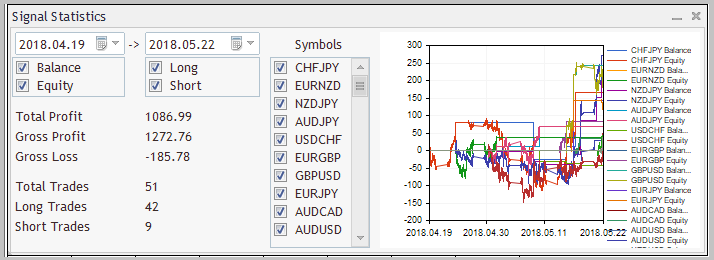We construct the graphic interface in class CStatisticsPanel (the inheriting class of class CAppDialog). We will use the instances of class CDatePicker to choose the analysis start/end dates. We join checkboxes for selecting the data to be displayed in 3 groups:

• Balance and Funds;
• Long and short positions; and
• List of symbols to be analyzed.

#### 3.1. Creating a graphic panel

To create the blocks of checkboxes, we will use the instances of class CCheckGroup. Text statistics will be displayed using the instances of class CLabel. The charts will be built using the instance of class CGraphic. And, of course, we will declare an instance of class COrdersCollection to have access to our statistics of orders.

```class CStatisticsPanel : public CAppDialog
{
private:
CDatePicker       StartDate;
CDatePicker       EndDate;
CLabel            Date;
CGraphic          Graphic;
CLabel            ShowLabel;
CCheckGroup       Symbols;
CCheckGroup       BalEquit;
CCheckGroup       Deals;
string            ar_Symbols[];
CLabel            TotalProfit;
CLabel            TotalProfitVal;
CLabel            GrossProfit;
CLabel            GrossProfitVal;
CLabel            GrossLoss;
CLabel            GrossLossVal;
//---
COrdersCollection Orders;

public:
CStatisticsPanel();
~CStatisticsPanel();
//--- main application dialog creation and destroy
virtual bool      Create(const long chart,const string name,const int subwin,const int x1,const int y1,const int x2,const int y2);
virtual void      Destroy(const int reason=REASON_PROGRAM);
//--- chart event handler
virtual bool      OnEvent(const int id,const long &lparam,const double &dparam,const string &sparam);

protected:
virtual bool      CreateLineSelector(const string name,const int x1,const int y1,const int x2,const int y2);
virtual bool      CreateDealsSelector(const string name,const int x1,const int y1,const int x2,const int y2);
virtual bool      CreateCheckGroup(const string name,const int x1,const int y1,const int x2,const int y2);
virtual bool      CreateGraphic(const string name,const int x1,const int y1,const int x2,const int y2);
//---
virtual void      Maximize(void);
virtual void      Minimize(void);
//---
virtual bool      UpdateChart(void);

};
```

In method Create, we will first call the relevant method of the parent class and then arrange all objects on their places and initialize the instance of the orders collection class. Upon initialization of each element, do not forget to assign initial values and add the object to the collection of control elements. Working with the basic class is elaborated in articles  and , so I will not go into a detailed description of the method, just present its code.

```bool CStatisticsPanel::Create(const long chart,const string name,const int subwin,const int x1,const int y1,const int x2,const int y2)
{
if(!CAppDialog::Create(chart,name,subwin,x1,y1,x2,y2))
return false;
//---
if(!TotalProfit.Create(m_chart_id,m_name+"Total Profit",m_subwin,5,80,115,95))
return false;
if(!TotalProfit.Text("Total Profit"))
return false;
return false;
//---
if(!TotalProfitVal.Create(m_chart_id,m_name+"Total Profit Value",m_subwin,135,80,250,95))
return false;
if(!TotalProfitVal.Text("0"))
return false;
return false;
//---
if(!GrossProfit.Create(m_chart_id,m_name+"Gross Profit",m_subwin,5,100,115,115))
return false;
if(!GrossProfit.Text("Gross Profit"))
return false;
return false;
//---
if(!GrossProfitVal.Create(m_chart_id,m_name+"Gross Profit Value",m_subwin,135,100,250,115))
return false;
if(!GrossProfitVal.Text("0"))
return false;
return false;
//---
if(!GrossLoss.Create(m_chart_id,m_name+"Gross Loss",m_subwin,5,120,115,135))
return false;
if(!GrossLoss.Text("Gross Loss"))
return false;
return false;
//---
if(!GrossLossVal.Create(m_chart_id,m_name+"Gross Loss Value",m_subwin,135,120,250,135))
return false;
if(!GrossLossVal.Text("0"))
return false;
return false;
//---
return false;
return false;
return false;
//---
return false;
return false;
return false;
//---
return false;
return false;
return false;
//---
return false;
return false;
return false;
//---
return false;
return false;
return false;
//---
return false;
return false;
return false;
//---
if(!Orders.Create())
return false;
//---
if(!ShowLabel.Create(m_chart_id,m_name+"Show Selector",m_subwin,285,8,360,28))
return false;
if(!ShowLabel.Text("Symbols"))
return false;
return false;
if(!CreateLineSelector("LineSelector",2,30,115,70))
return false;
if(!CreateDealsSelector("DealsSelector",135,30,250,70))
return false;
if(!CreateCheckGroup("CheckGroup",260,30,360,ClientAreaHeight()-5))
return false;
//---
if(!Date.Create(m_chart_id,m_name+"->",m_subwin,118,8,133,28))
return false;
if(!Date.Text("->"))
return false;
return false;
//---
if(!StartDate.Create(m_chart_id,m_name+"StartDate",m_subwin,5,5,115,28))
return false;
return false;
//---
if(!EndDate.Create(m_chart_id,m_name+"EndDate",m_subwin,135,5,250,28))
return false;
return false;
//---
StartDate.Value(Orders.FirstOrder());
EndDate.Value(Orders.LastOrder());
//---
if(!CreateGraphic("Chraphic",370,5,ClientAreaWidth()-5,ClientAreaHeight()-5))
return false;
//---
UpdateChart();
//---
return true;
}
```

An observant reader may notice that the chart created has not been added to the collection of control elements. This is because object CGraphic is not inherited from class CWnd, whereas you only may only add to the collection the CWnd-inheriting objects. Therefore, we will have to re-write the functions of minimizing/deploying the panel.
Upon initialization of all objects, we will call the chart updating function.

#### 3.2. Chart creation function

Let us gloss over chart creation function CreateGraphic. In its parameters, it gets the name of the object created and the coordinates of the chart location. In the beginning of the function, the chart is created (calling function Create of class CGraphic). Since class CGraphic is not inherited from class CWnd and we will not be able to add it to the collection of control elements of the panel, the chart coordinates will be immediately shifted in accordance with the client area location.

```bool CStatisticsPanel::CreateGraphic(const string name,const int x1,const int y1,const int x2,const int y2)
{
if(!Graphic.Create(m_chart_id,m_name+name,m_subwin,ClientAreaLeft()+x1,ClientAreaTop()+y1,ClientAreaLeft()+x2,ClientAreaTop()+y2))
return false;
```

Then we have to create the instances of class CCurve for each curve displayed in the chart. For this purpose, we will first get the list of symbols used from the instance of class COrdersCollection. Then we will create in the loop the curves of balance and funds for each symbol, having initialized them with an empty array. Upon creation, we hide the lines in the chart until the data is obtained.

```   int total=Orders.Symbols(ar_Symbols);
CColorGenerator ColorGenerator;
double array[];
ArrayFree(array);
for(int i=0;i<total;i++)
{
//---
curve.Visible(false);
curve.Visible(false);
}
```

Upon creating the curves, we disable auto-scaling the abscissa scale and specify for it the property of displaying as dates. We also specify the sizes of displaying the curve captions and display the chart on the screen.

```   CAxis *axis=Graphic.XAxis();
axis.AutoScale(false);
axis.Type(AXIS_TYPE_DATETIME);
axis.ValuesDateTimeMode(TIME_DATE);
Graphic.HistorySymbolSize(20);
Graphic.HistoryNameSize(10);
Graphic.HistoryNameWidth(60);
Graphic.CurvePlotAll();
Graphic.Update();
//---
return true;
}
```

#### 3.3. Chart and statistical data updating method

We are going to use method UpdateChart for updating information on the signal. At the function beginning, we prepare variables and arrays for collecting the data.

```bool CStatisticsPanel::UpdateChart(void)
{
double balance[];
double equity[];
double time[];
double total_profit=0, total_loss=0;
int total_long=0, total_short=0;
CCurve *Balance, *Equity;
```

Then we get the start/end dates of the period to be analyzed.

```   datetime start=StartDate.Value();
datetime end=EndDate.Value();
```

Check whether the marks show the statistics of long and short positions.

```   int deals=-2;
if(Deals.Check(0))
else
deals=(Deals.Check(1) ? POSITION_TYPE_SELL : -2);
```

Upon preparing the initial data in the loop for each symbol, we will update the timeseries by calling function GetTimeSeries already familiar to us. Before calling the method, we check the tick in the checkbox of the relevant symbol. If it is not there, the method is not called and the curves are hidden. Upon successfully getting the timeseries, we will update the date for the curves of balance and funds and precheck the ticks in the relevant checkboxes. If it is not ticked, the curve will be hidden in the chart.

```   int total=ArraySize(ar_Symbols);
for(int i=0;i<total;i++)
{
Balance  =  Graphic.CurveGetByIndex(i*2);
Equity   =  Graphic.CurveGetByIndex(i*2+1);
double profit,loss;
{
if(BalEquit.Check(0))
{
Balance.Update(time,balance);
Balance.Visible(true);
}
else
Balance.Visible(false);
if(BalEquit.Check(1))
{
Equity.Update(time,equity);
Equity.Visible(true);
}
else
Equity.Visible(false);
total_profit+=profit;
total_loss+=loss;
}
else
{
Balance.Visible(false);
Equity.Visible(false);
}
}
```

As the next step, we specify for the chart the start/end dates of the period to be analyzed, as well as the grid size. Update the chart.

```   CAxis *axis=Graphic.XAxis();
axis.Min((double)start);
axis.Max((double)end);
axis.DefaultStep((end-start)/5);
if(!Graphic.Redraw(true))
return false;
Graphic.Update();
```

In conclusion of the method, we update information in text marks to display statistics for the signal.

```   if(!TotalProfitVal.Text(DoubleToString(total_profit+total_loss,2)))
return false;
if(!GrossProfitVal.Text(DoubleToString(total_profit,2)))
return false;
if(!GrossLossVal.Text(DoubleToString(total_loss,2)))
return false;
return false;
return false;
return false;
//---
return true;
}
```

#### 3.4. "Animating" the panel

To "animate" the m=panel, we will have to build an event handler for the actions with objects. What are possible events the program will have to handle?

First of all, this is changing the date of starting or ending the period to be analyzed and changing the state of checkboxes that control collecting the statistics and displaying the curves of balance and funds. We should not forget about our trick: We will have to handle a user event created where it is impossible to load the quotes history for one of the symbols analyzed. When any of those events occurs, it is sufficient to call data updating method UpdateChart. As a result, the event handling method will appear as:

```EVENT_MAP_BEGIN(CStatisticsPanel)
ON_EVENT(ON_CHANGE,Symbols,UpdateChart)
ON_EVENT(ON_CHANGE,BalEquit,UpdateChart)
ON_EVENT(ON_CHANGE,Deals,UpdateChart)
ON_EVENT(ON_CHANGE,StartDate,UpdateChart)
ON_EVENT(ON_CHANGE,EndDate,UpdateChart)
ON_NO_ID_EVENT(1222,UpdateChart)
EVENT_MAP_END(CAppDialog)
```

Along with the above methods, we have also changed the methods of minimizing/deploying the panel, i.e., we have added the chart hiding/showing function in them. You can find attached the complete code of the class and methods.

### 4. Creating an indicator to analyze the signal

I propose to unify all the above as an indicator. This will allow us to create a graphical panel in a subwindow without involving the chart itself.

All the functionality of our program is hidden in class CStatisticsPanel. Therefore, to create an indicator, it is sufficient to create an instance of this class in our program. Initializing the class in function OnInit.

```int OnInit()
{
//---
long chart=ChartID();
int subwin=ChartWindowFind();
IndicatorSetString(INDICATOR_SHORTNAME,"Signal Statistics");
//---
Dialog=new CStatisticsPanel;
if(CheckPointer(Dialog)==POINTER_INVALID)
{
ChartIndicatorDelete(chart,subwin,"Signal Statistics");
return INIT_FAILED;
}
if(!Dialog.Create(chart,"Signal Statistics",subwin,0,0,0,250))
{
ChartIndicatorDelete(chart,subwin,"Signal Statistics");
return INIT_FAILED;
}
if(!Dialog.Run())
{
ChartIndicatorDelete(chart,subwin,"Signal Statistics");
return INIT_FAILED;
}
//---
return(INIT_SUCCEEDED);
}
```

We leave function OnCalculate empty, since the program will not react to the next tick coming in. It remains for us just to add calling the relevant methods in function OnDeinit and OnChartEvent.

```void OnChartEvent(const int id,
const long &lparam,
const double &dparam,
const string &sparam)
{
//---
Dialog.ChartEvent(id,lparam,dparam,sparam);
}
//+------------------------------------------------------------------+
//|                                                                  |
//+------------------------------------------------------------------+
void OnDeinit(const int reason)
{
Dialog.Destroy(reason);
delete Dialog;
}
```

Having compiled the indicator, we will only need upload to the terminal chart the statistics of the selected signal and attach our indicator to one of the charts. Now we can study and analyze trades. There is a finer point: We have not filtered the charts for analysis in our program. Therefore, the indicator will collect statistics from all charts opened in the terminal. In order to avoid mixing the indicator trades with any other trades in the terminal, I recommend to close all charts before loading the signal trade history.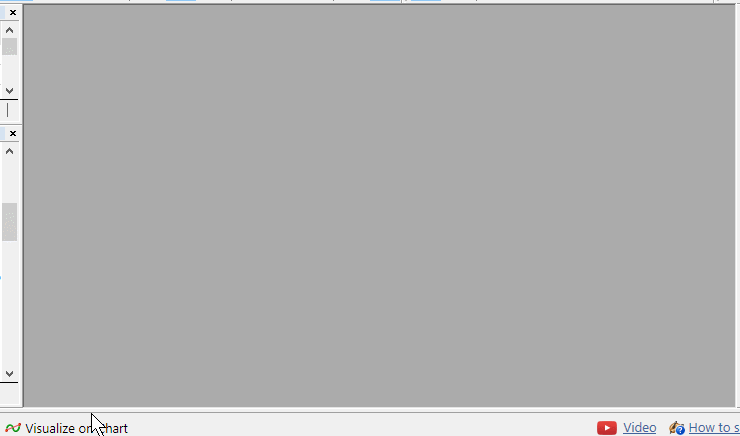You can find attached the complete code of the program.

### Conclusion

We have built an indicator analyzing trades by the marks in charts. This technology may be used for various purposes, such as in choosing a signal or optimizing your own strategy. For example, this will allow you to identify symbols, for which our strategy does not work, in order not to use it on those symbols in future.

### References

#
Name
Type
Description
1 Order.mqh  Class library  Class for storing information on a trade
2 OrdersCollection.mqh  Class library  Trades collection class
3 StatisticsPanel.mqh  Class library  Graphic interface class
4 SignalStatistics.mq5  Indicator  Code of an indicator for analyzing trades

Translated from Russian by MetaQuotes Ltd.
Original article: https://www.mql5.com/ru/articles/4751

Attached files |
MQL5.zip (470.5 KB)

#### Other articles by this author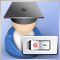Expert Advisor featuring GUI: Creating the panel (part I)
Despite the fact that many traders still prefer manual trading, it is hardly possible to completely avoid the automation of routine operations. The article shows an example of developing a multi-symbol signal Expert Advisor for manual trading.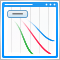Improving Panels: Adding transparency, changing background color and inheriting from CAppDialog/CWndClient
In this article, we continue studying the use of CAppDialog. Now we will learn how to set color for the background, borders and header of the dialog box. Also, this article provides a step-by-step description of how to add transparency for an application window when dragging it within the chart. We will consider how to create child classes of CAppDialog or CWndClient and analyze new specifics of working with controls. Finally, we will review new Projects from a new perspective.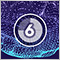Deep Neural Networks (Part VI). Ensemble of neural network classifiers: bagging
The article discusses the methods for building and training ensembles of neural networks with bagging structure. It also determines the peculiarities of hyperparameter optimization for individual neural network classifiers that make up the ensemble. The quality of the optimized neural network obtained in the previous article of the series is compared with the quality of the created ensemble of neural networks. Possibilities of further improving the quality of the ensemble's classification are considered.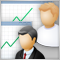Social Trading. Can a profitable signal be made even better?
Most subscribers choose a trade signal by the beauty of the balance curve and by the number of subscribers. This is why many today's providers care of beautiful statistics rather than of real signal quality, often playing with lot sizes and artificially reducing the balance curve to an ideal appearance. This paper deals with the reliability criteria and the methods a provider may use to enhance its signal quality. An exemplary analysis of a specific signal history is presented, as well as methods that would help a provider to make it more profitable and less risky.